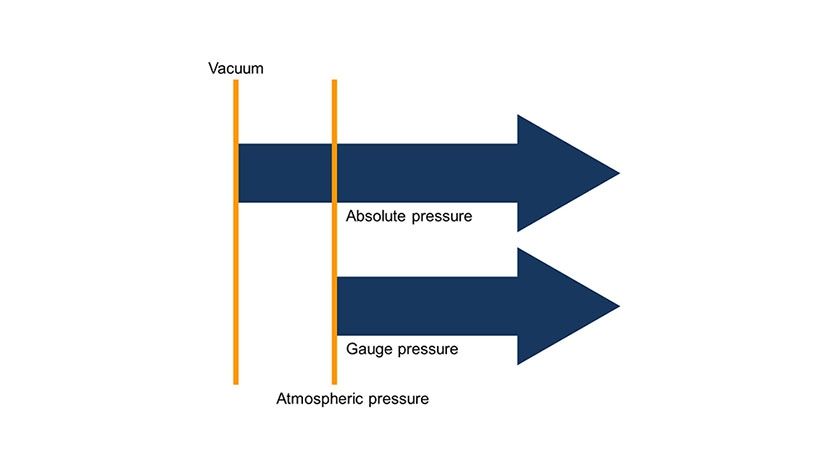Again and again, we are asked the question about the difference between an absolute pressure measurement and a gauge pressure measurement. To answer this question it helps to look at the definition of gauge pressure and absolute pressure. The difference between the two measurements is then explained relatively simply and thus also the choice of the appropriate measurement.

## Definition of absolute pressure

Absolute pressure is a pressure that is relative to the zero pressure in the empty, air-free space of the universe. This reference pressure is the ideal or absolute vacuum. It is denoted with the subscript “abs”: Pabs.

## Definition of gauge pressure

The gauge pressure is defined as the difference between an absolute pressure (Pabs) and the prevailing atmospheric pressure (Pamb). It is denoted with the subscript “e”: Pe and is calculated as follows: Pe = Pabs – Pamb.

## Absolute vs gauge pressure measurement

The difference between the two measurements is relatively easily clarified: in a gauge pressure measurement, it is always the difference from the current ambient pressure that is measured. However, this pressure changes with the weather and the height above sea level. An absolute pressure measurement measures the difference from the ideal or absolute vacuum. This is why this measurement is independent of environmental influences such as weather or altitude. Which measurement is now the right one?

In practice, the two measurements can be differentiated as follows: in most cases, the measuring task is to determine the gauge pressure. This is why this type of sensor is most widely used. However, if a gauge pressure sensor is used in an application in which the actual measuring task is to measure the absolute pressure, the following additional errors must be expected:

• +/- 30 mbar caused by changes in weather
• up to 200 mbar when changing the location (e.g. from sea level to 2,000 m)

Depending on the measuring range, these errors can be substantial (e.g. in pneumatics at a measuring range of 1 bar) or negligible (in hydraulics at 400 bar).

Note
If you are uncertain whether your measuring task needs an absolute pressure or gauge pressure measurement, simply contact us – we’ll be glad to assist you.

1 comment
1.Thanks for such simple clarification.